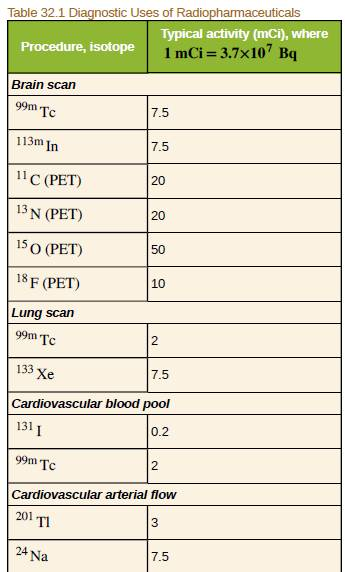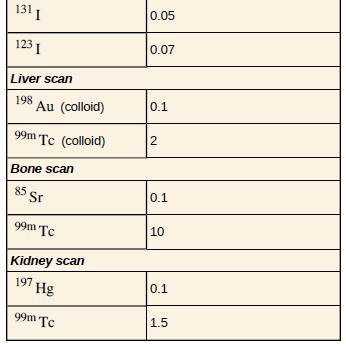Chapter 32, Problem 5PE

Chapter
Section
Textbook Problem

Table 32.1 indicates that 7.50 mCi of 99mTc is used in a brain scan. What is the mass of technetium?To determine

The mass of the technetium.

Explanation

Given info:

The activity is

A=7.50m·Ci

Formula used:

The mass can be found as

m=NNa×molarmass

Calculation:

The activity

A=7.50m·Ci=7.50×3.70×107BqA=2.78×108Bq

Activity is defined as

A=Nln2T 1 2N=AT1/2ln2

Substituting the values

N=(2

Still sussing out bartleby?

Check out a sample textbook solution.

See a sample solution

The Solution to Your Study Problems

Bartleby provides explanations to thousands of textbook problems written by our experts, many with advanced degrees!

Get Started

Find more solutions based on key concepts